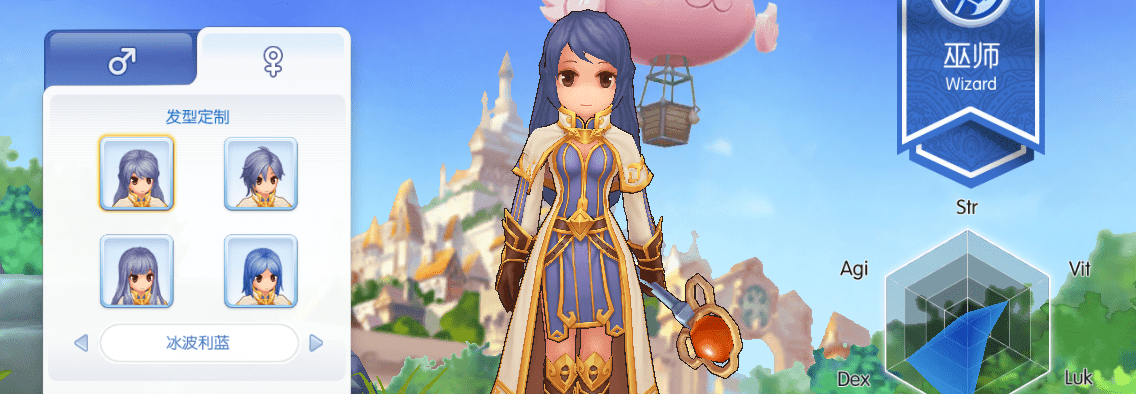# 属性雷达图UGUI渲染器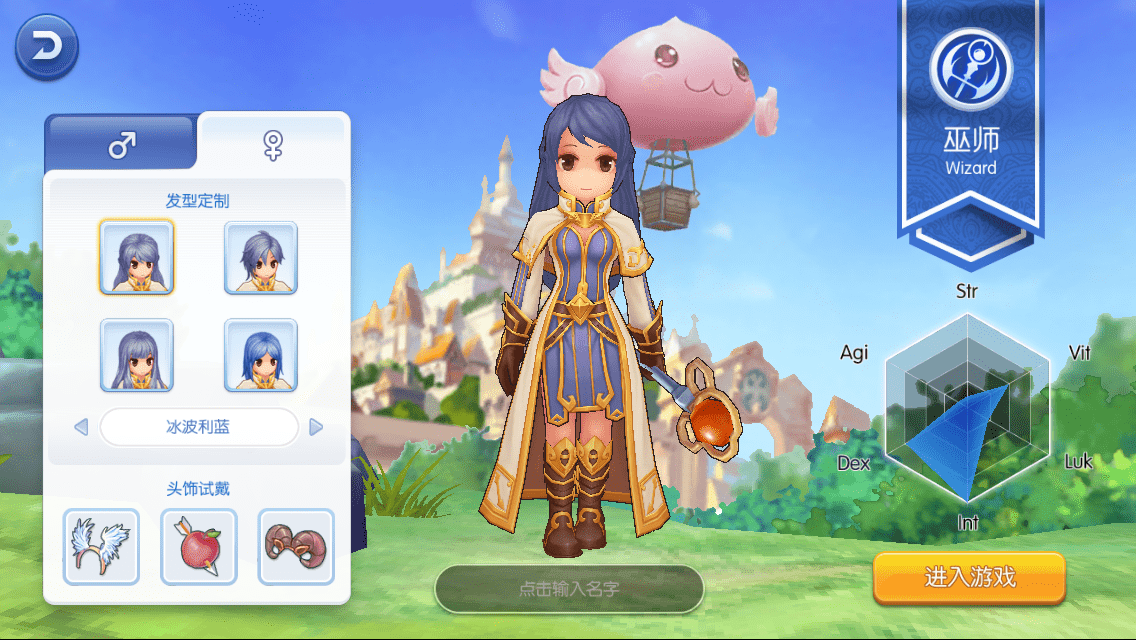MMORPG手游《仙境传说RO：守护永恒的爱》中创建角色页面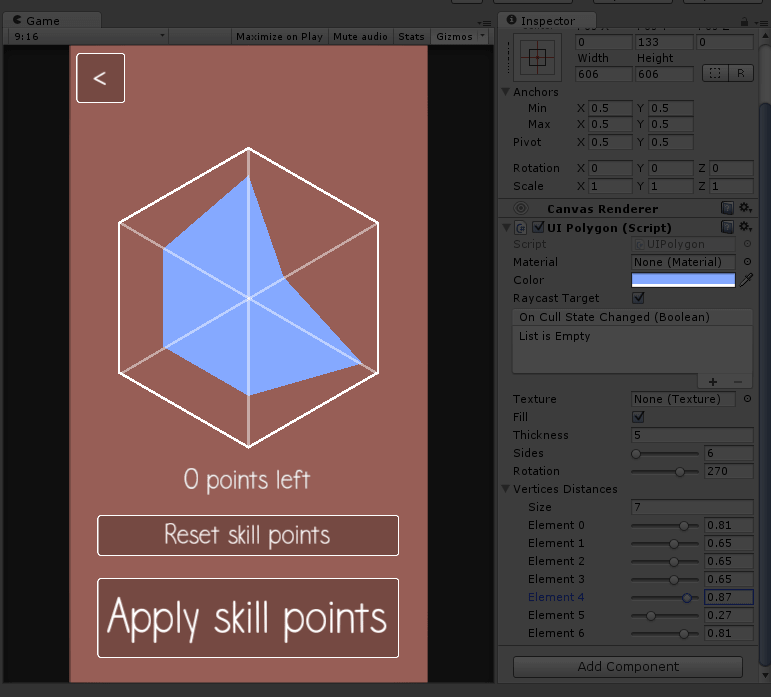## 使用方法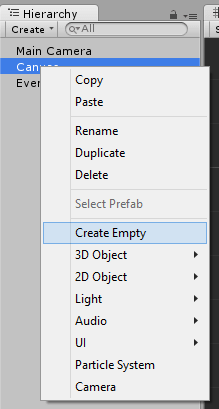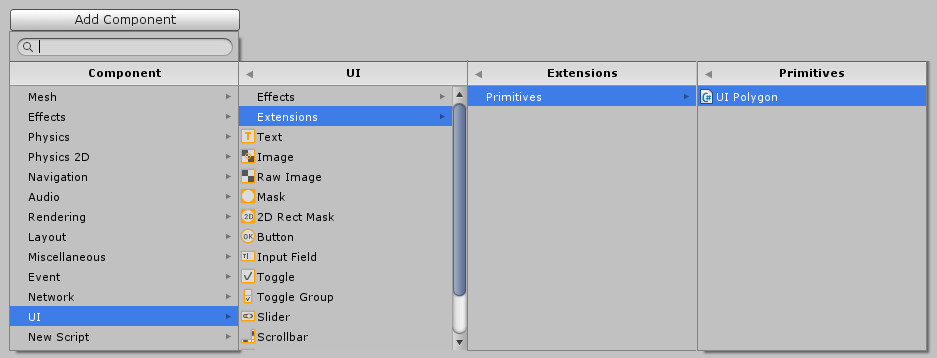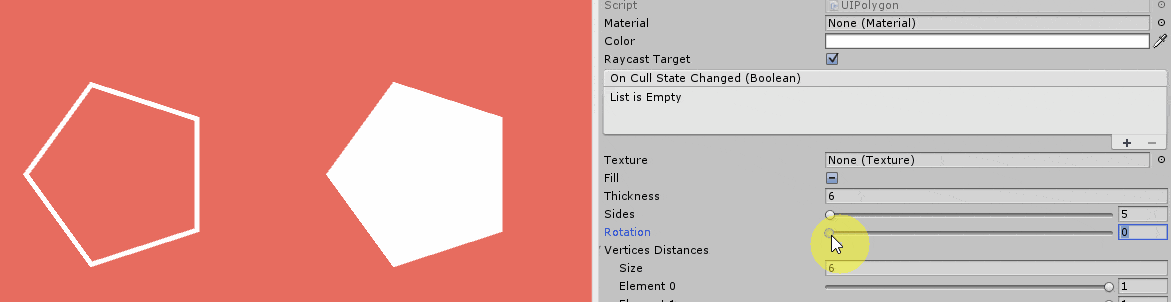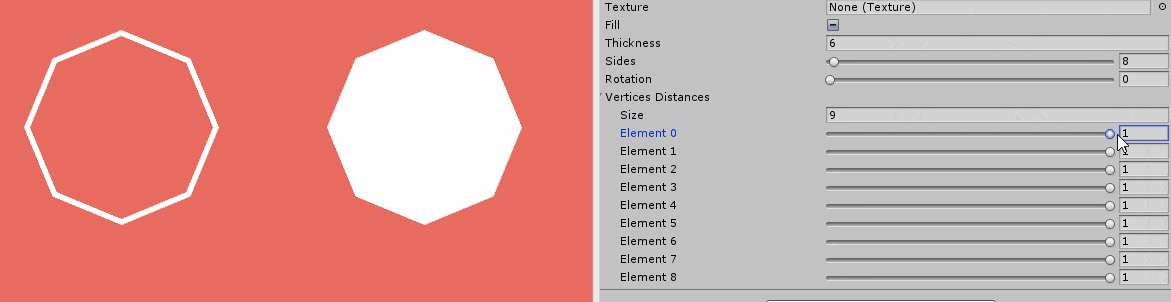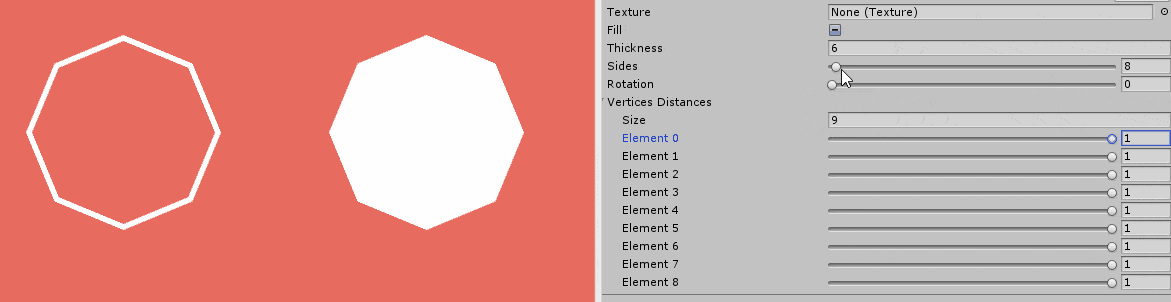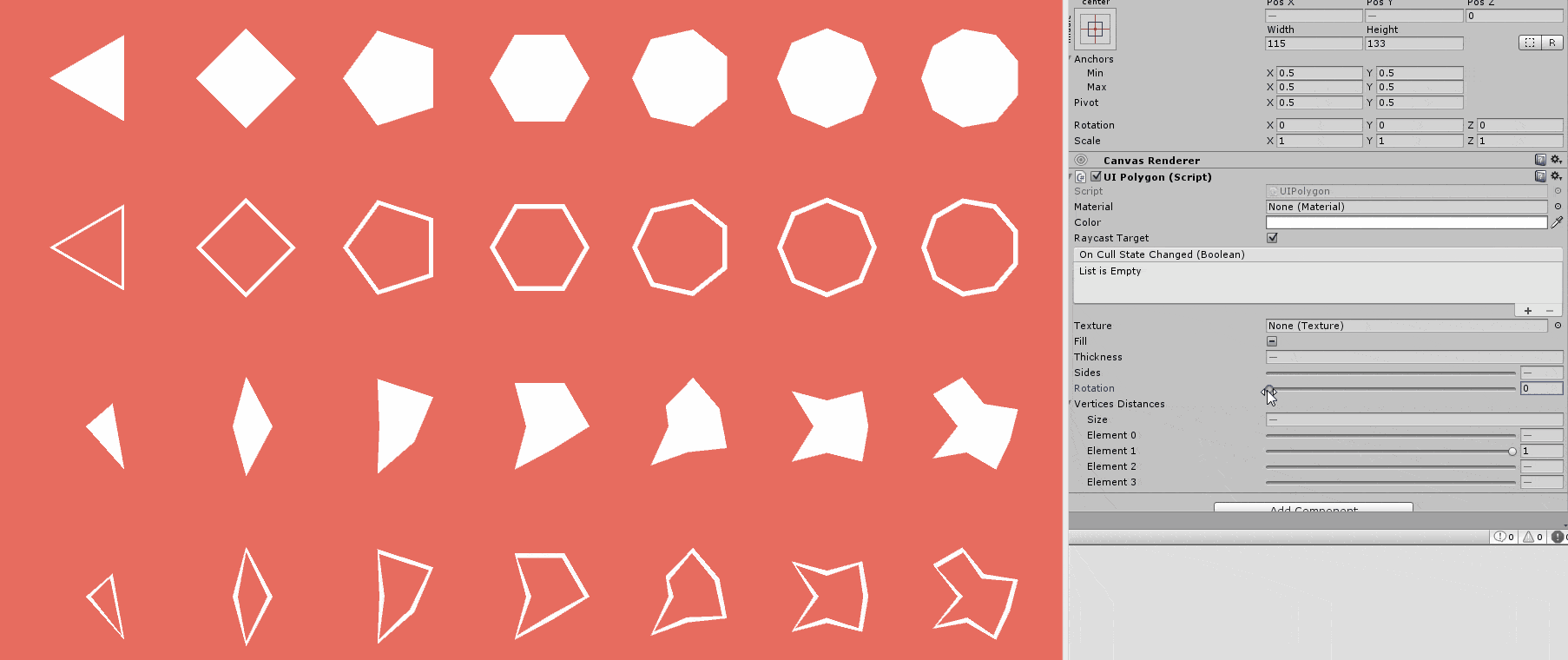## 实现方式

``````void Update()
{
size = rectTransform.rect.width;
if (rectTransform.rect.width > rectTransform.rect.height)
size = rectTransform.rect.height;
else
size = rectTransform.rect.width;
thickness = (float)Mathf.Clamp(thickness, 0, size / 2);
}
``````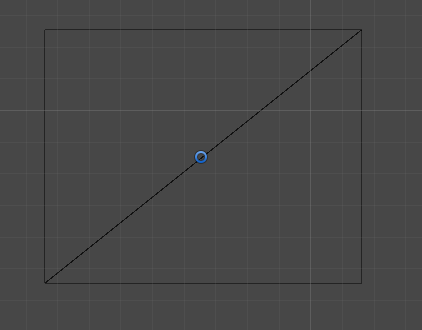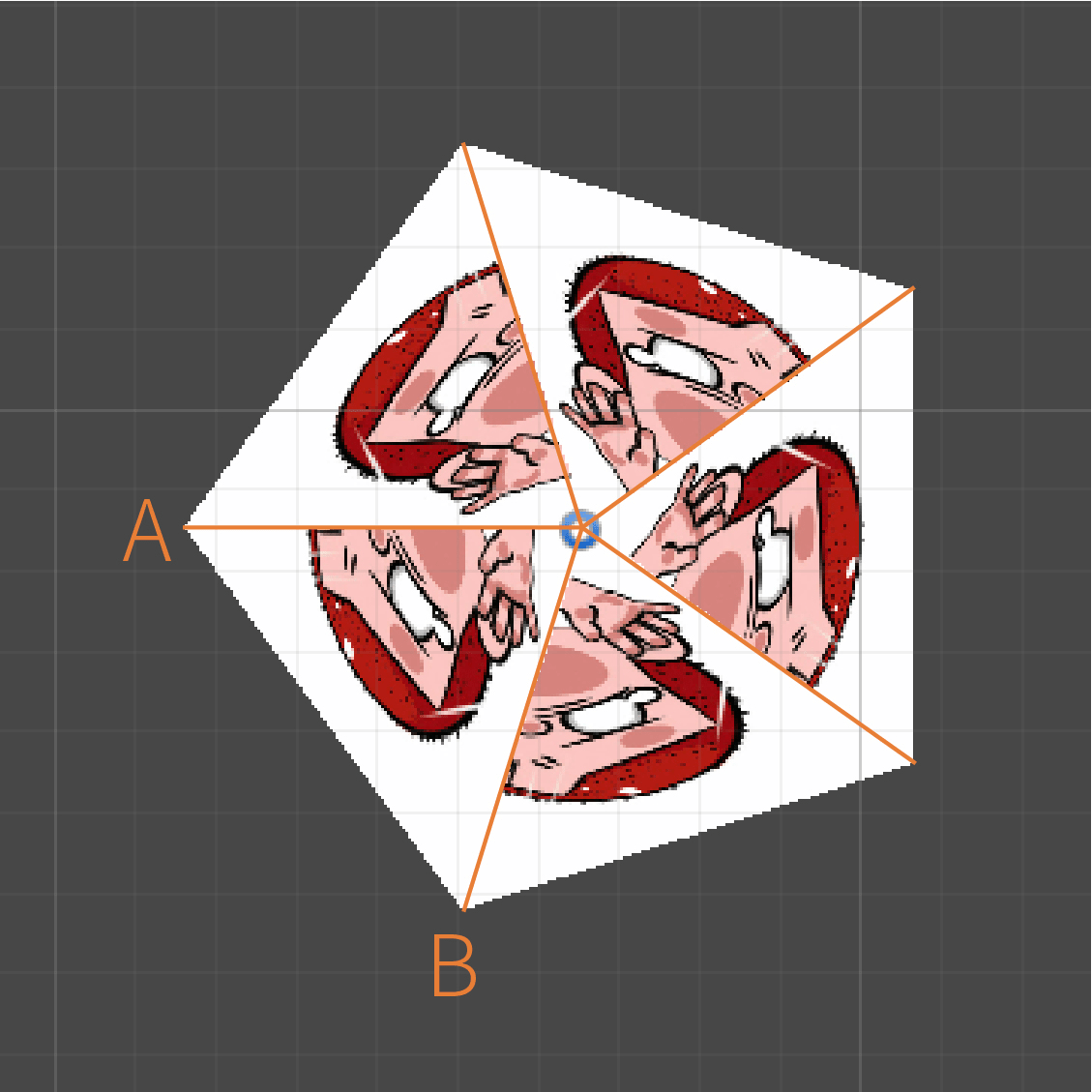``````Vector2 prevX = Vector2.zero;
Vector2 prevY = Vector2.zero;
``````

``````float degrees = 360f / sides;
float outer = -rectTransform.pivot.x * size * VerticesDistances[i];
pos1 = new Vector2(outer * c, outer * s);
``````

``````pos0 = prevX;
pos1 = new Vector2(outer * c, outer * s);
pos2 = Vector2.zero;
pos3 = Vector2.zero;
prevX = pos1;
prevY = pos2;
vh.AddUIVertexQuad(SetVbo(new[] { pos0, pos1, pos2, pos3 }, new[] { uv0, uv1, uv2, uv3 }));
``````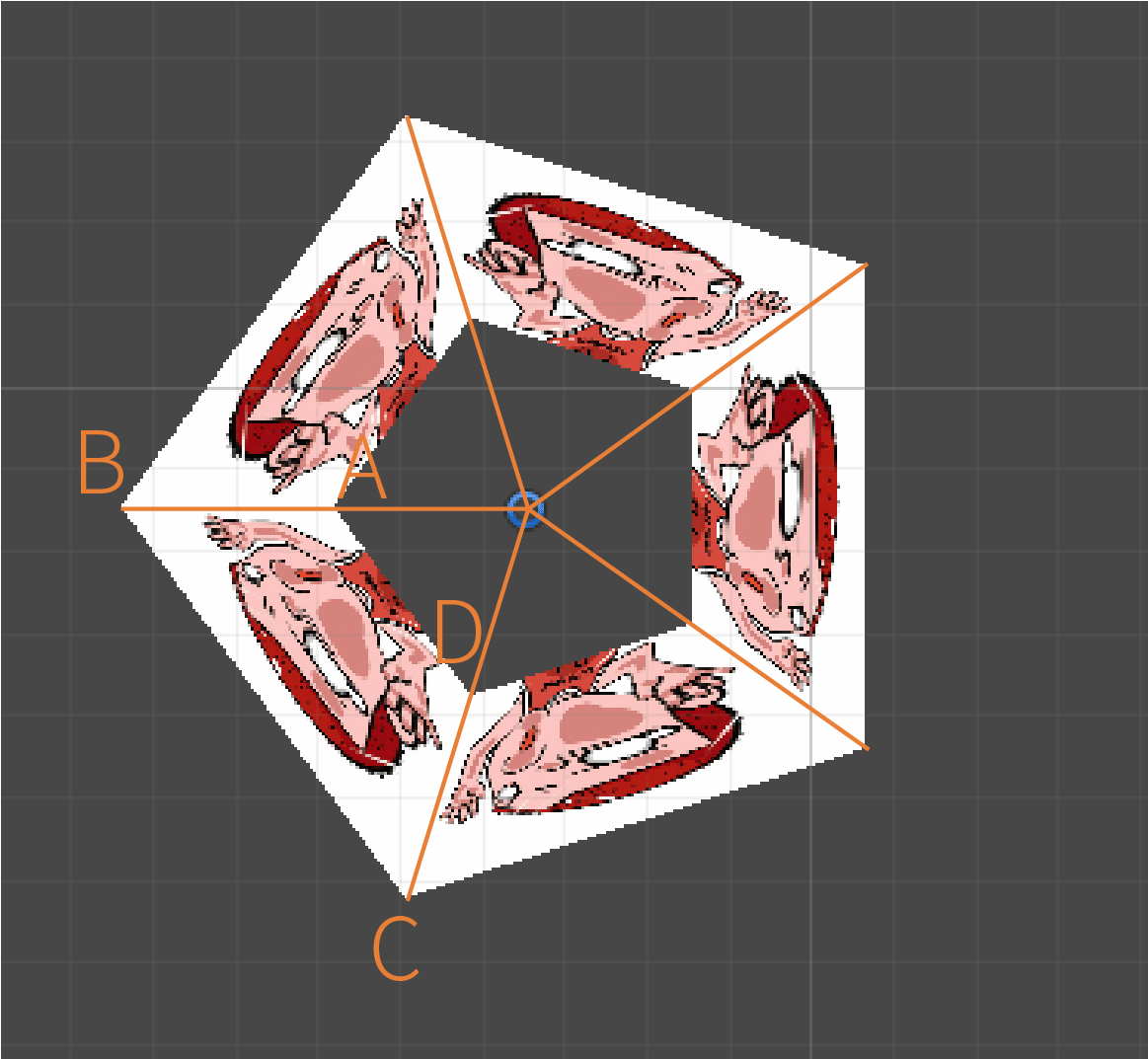``````float inner = -rectTransform.pivot.x * size * VerticesDistances[i] + thickness;
pos2 = new Vector2(inner * c, inner * s);
pos3 = prevY;
``````

``````public void DrawPolygon(int _sides)
{
sides = _sides;
VerticesDistances = new float[_sides + 1];
for (int i = 0; i < _sides; i++) VerticesDistances[i] = 1;
rotation = 0;
}
public void DrawPolygon(int _sides, float[] _VerticesDistances)
{
sides = _sides;
VerticesDistances = _VerticesDistances;
rotation = 0;
}
public void DrawPolygon(int _sides, float[] _VerticesDistances, float _rotation)
{
sides = _sides;
VerticesDistances = _VerticesDistances;
rotation = _rotation;
}
``````

## 性能测评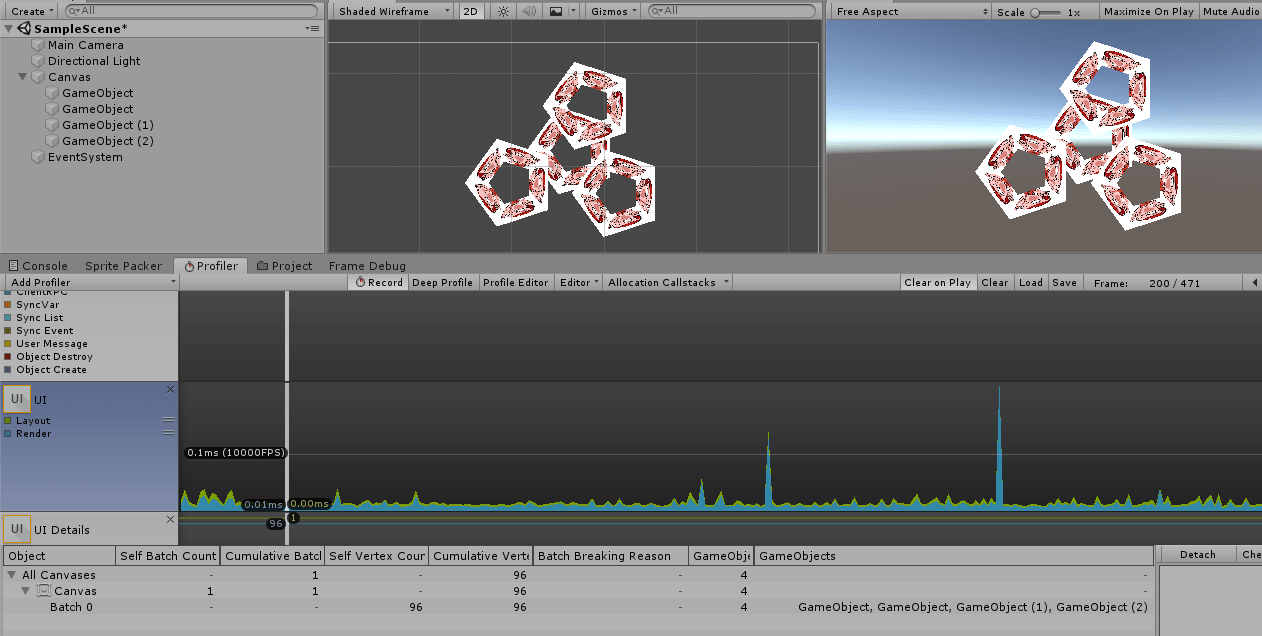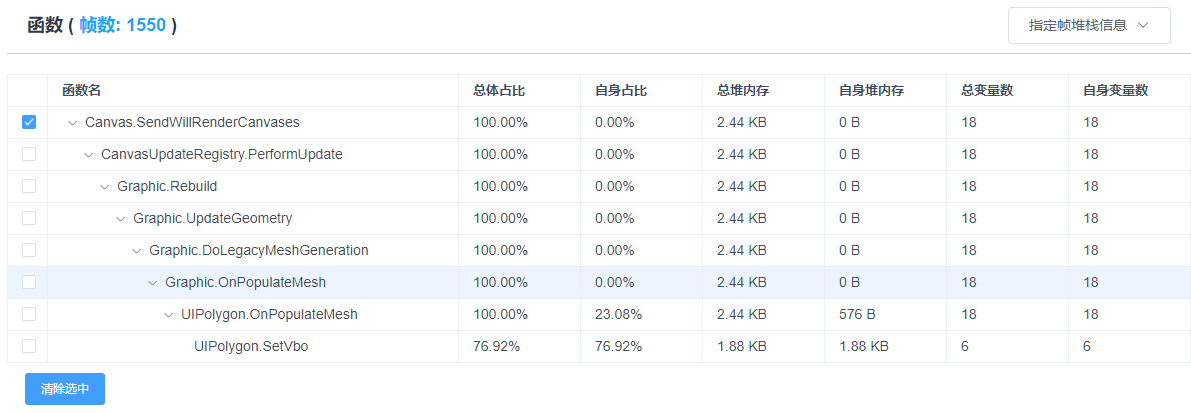``````UIVertex[] vbo = new UIVertex;
``````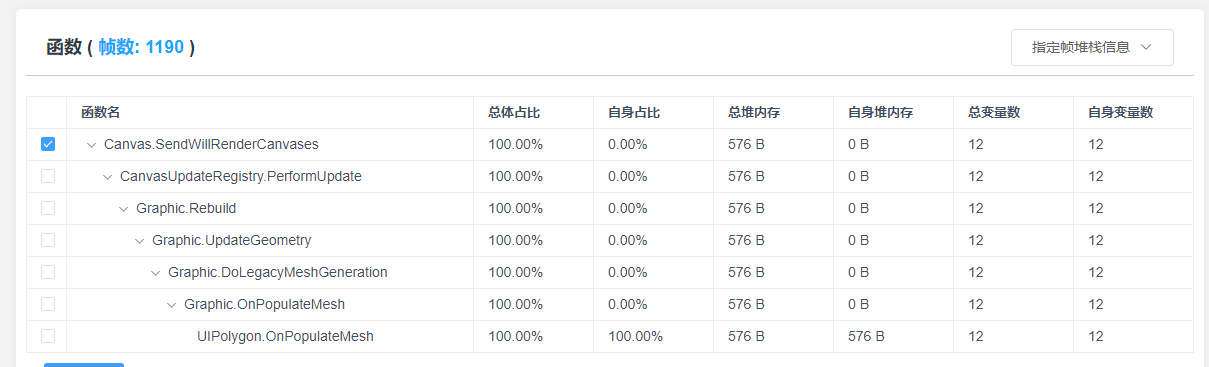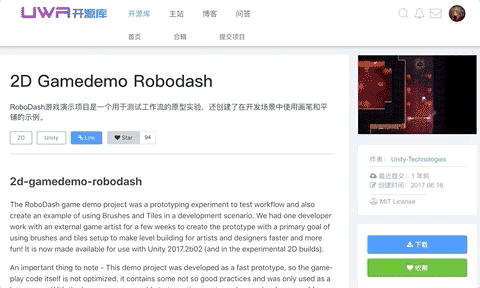【博物纳新】是UWA旨在为开发者推荐新颖、易用、有趣的开源项目，帮助大家在项目研发之余发现世界上的热门项目、前沿技术或者令人惊叹的视觉效果，并探索将其应用到自己项目的可行性。很多时候，我们并不知道自己想要什么，直到某一天我们遇到了它。

•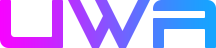Bonnie 发表在 2019年09月05日 回复

很棒！